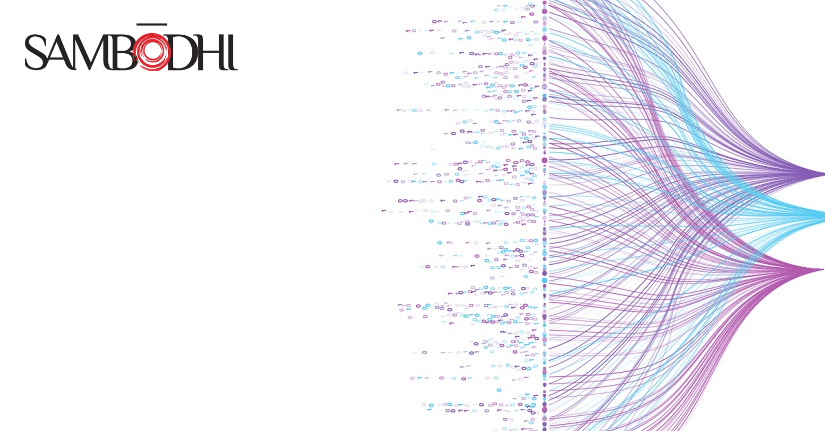# Quantile Regression

> > > Quantile Regression
Posted by: Kultar Singh
Category: Data ScienceQuantile regression is a popular regression model used extensively to assess treatment heterogeneity. It is an extension of a regular regression model and is commonly used in quantitative modelling.

#### Understanding Quantile Regression

Standard linear regression employs the least squares method to determine the result variable’s conditional mean over multiple regressor values. The conditional mean characterizes the relationship between regressors and the outcome variable based on linear regression, which provides a partial view of the relationship. Sometimes we may be interested in defining it at different points in the conditional distribution of outcome variables. Quantile regression can be described as an extension of standard linear regression that determines the conditional median of the outcome variable.

#### Defining Quantiles

But before going further, let’s revisit quantiles as a concept. Quantiles are points in a distribution that signify the rank order of values. The median is the middle quantile or the 50th percentile of the distribution. Hence, quantile regression is also termed the median regression model. While the median regression estimates the median or the 50th percentile of the dependent variable, this regression technique can also estimate other percentiles like the 25th or the 75th.

It is well known that the median is not affected by outliers, and quantile regression can be used effectively in the case of outlier values. Moreover, it is used increasingly for subgroup analysis, wherein the differential in outcome variable like income across different strata of the percentile of the population and the factors resulting in the difference may be researched.

#### Benefits of quantile regression

The benefit of quantile regression is that it permits understanding relationships between variables that are not part of the conditional means of the response. It helps study an outcome’s different quantiles and compare different levels of exposure to these quantiles.

#### Regression and Quantile regression

A multivariable regression model can be a powerful research method with numerous applications. Although linear regression can be used to describe what is expected to be the value of an outcome that is continuous, based on the covariates of the model, quantile regression is a method to evaluate the whole range of a continuous response or one specific quantity of the response across groups. While linear regression uses the method of least squares to calculate the mean of the outcome variable, quantile regression estimates the conditional median of the outcome variable. In other words, the quantile regression estimates the conditional distribution of a specific percentile’s dependent variable based on explanatory variables.

#### When to utilize quantile regression

Quantile regression is a great tool when the objective is to estimate the regression with outliers, and the key assumption of linear regression is not satisfied. It is used to estimate the median or any quantile. It is also a great tool for performing sub-group analysis.

#### Using quantile regression for heterogeneous treatment effects

The heterogeneity of data is a regular issue in research. A substantial part of the literature on programme assessment explores heterogeneous treatment effects utilizing subgroup analysis. If data is heterogeneous, an equal amount of variation in the data to construct an appropriate model for the investigated issues is impossible to assume. The standard least square regression method doesn’t consider the different variations, which could result in an inaccurate estimation of the causality between variables. In contrast, quantile regression can effectively address this issue by detecting the relation between variables at various levels.

Kultar Singh – Chief Executive Officer, SambodhiAuthor: Kultar Singh